Open in App
Not now

# Minimum operations required to make all elements of Array less than equal to 0

• Difficulty Level : Medium
• Last Updated : 20 Feb, 2023

Given an array arr[] consisting of N positive numbers, the task is to find the minimum number of operations required to make all elements of the array less than or equal to 0. In each operation, one has to pick the minimum positive element from the array and subtract all the elements of the array from that number.

Examples:

Input: arr[] = {1, 2, 4, 2, 2, 5, 6}
Output: 5
Explanation: The explanation is mentioned in the diagram below: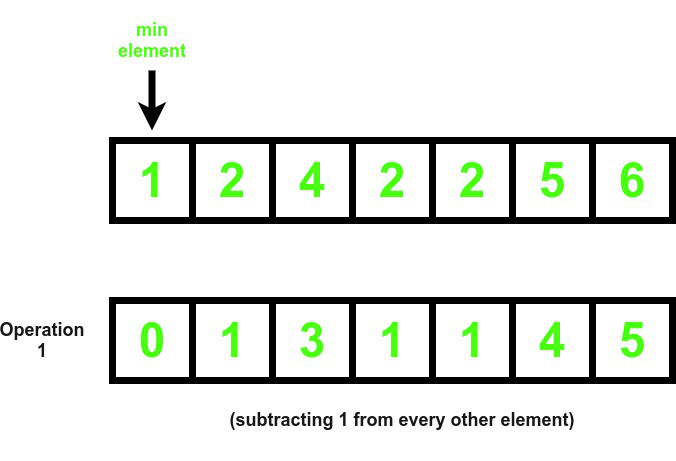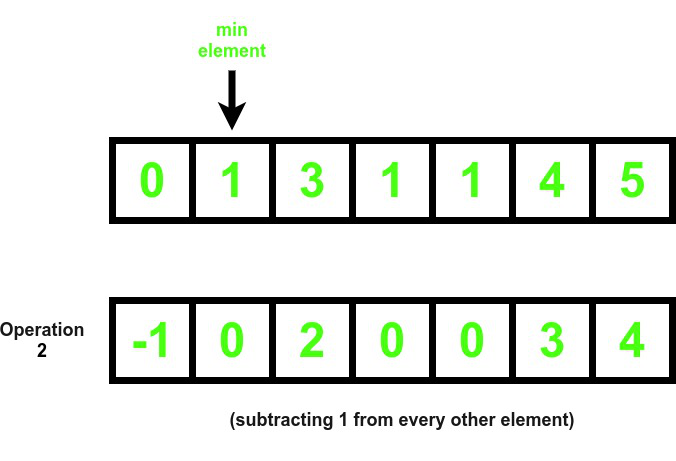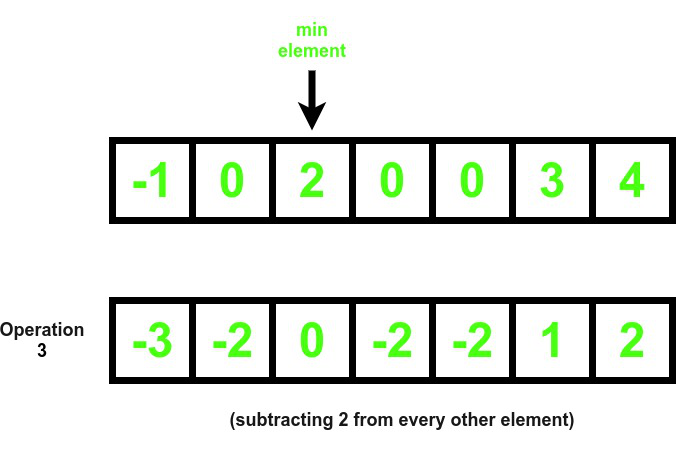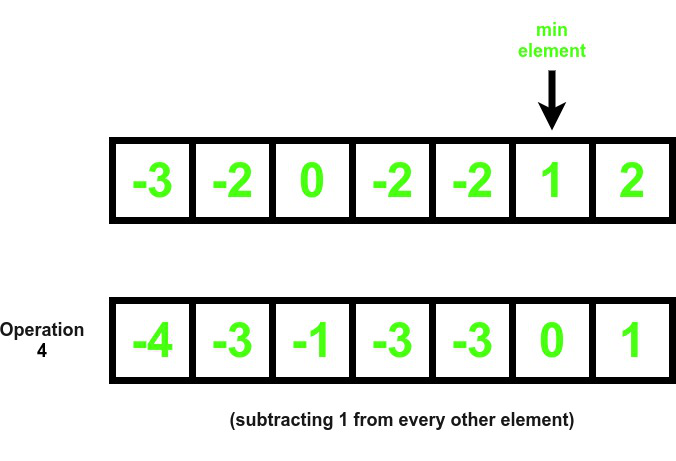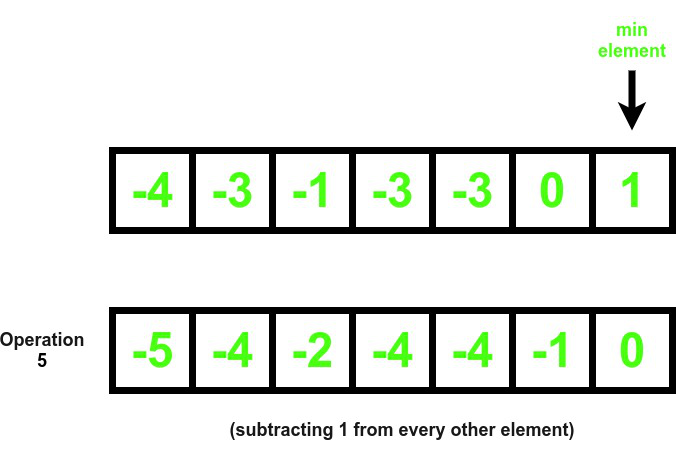The resulting array has met the criteria as all it’s elements are either less than or equal to 0

Input: arr[] = {1, 2, 3}
Output: 3

Naive Approach: The simplest approach to solve the problem is to Traverse the array while all the elements of the array are not less than or equal to 0 and find the minimum non-zero positive element and subtract that element from the whole array.

Time Complexity: O(N2
Auxiliary Space: O(1)

Efficient Approach: The above approach can be optimized further by observing that the answer will be the number of non-zero distinct elements in the array. Follow the steps below to solve the problem:

• Initialize a hash-map say m that stores the unique elements present in the array.
• Iterate in the range [0, N-1] using the variable i and mark m[arr[i]] as 1.
• Print the value of m.size() as the answer.

Below is the implementation of the above approach:

## C++

 `// C++ Program for the above approach` `#include ` `using` `namespace` `std;`   `// Function to find minimum number of` `// non-zero elements that has to be subtracted` `// such that all the elements are less than or 0` `int` `distinct(vector<``int``> arr)` `{` `    ``// Hash map to mark elements true` `    ``// that are present in the array` `    ``map<``int``, ``bool``> m;`   `    ``// Traverse the array` `    ``for` `(``int` `i = 0; i < arr.size(); i++) {` `        ``// Mark arr[i] true` `        ``m[arr[i]] = ``true``;` `    ``}`   `    ``// Finally, return the size of hashmap` `    ``return` `m.size();` `}`   `// Driver Code` `int` `main()` `{`   `    ``// Given Input` `    ``vector<``int``> arr = { 1, 2, 4, 2, 2, 5, 6 };`   `    ``// Function Call` `    ``int` `ans = distinct(arr);` `    ``cout << ans << endl;` `    ``return` `0;` `}`

## Java

 `// Java program for the above approach` `import` `java.util.HashMap;`   `class` `GFG{` `    `  `// Function to find minimum number of` `// non-zero elements that has to be subtracted` `// such that all the elements are less than or 0` `public` `static` `int` `distinct(``int``[] arr) ` `{` `    `  `    ``// Hash map to mark elements true` `    ``// that are present in the array` `    ``HashMap m = ``new` `HashMap();`   `    ``// Traverse the array` `    ``for``(``int` `i = ``0``; i < arr.length; i++) ` `    ``{` `        `  `        ``// Mark arr[i] true` `        ``m.put(arr[i], ``true``);` `    ``}`   `    ``// Finally, return the size of hashmap` `    ``return` `m.size();` `}`   `// Driver Code` `public` `static` `void` `main(String args[])` `{` `    `  `    ``// Given Input` `    ``int``[] arr = { ``1``, ``2``, ``4``, ``2``, ``2``, ``5``, ``6` `};`   `    ``// Function Call` `    ``int` `ans = distinct(arr);` `    `  `    ``System.out.println(ans);` `}` `}`   `// This code is contributed by gfgking`

## Python3

 `# Python 3 Program for the above approach`   `# Function to find minimum number of` `# non-zero elements that has to be subtracted` `# such that all the elements are less than or 0` `def` `distinct(arr):`   `    ``# Hash map to mark elements true` `    ``# that are present in the array` `    ``m ``=` `{}`   `    ``# Traverse the array` `    ``for` `i ``in` `range``(``len``(arr)):` `        ``# Mark arr[i] true` `        ``m[arr[i]] ``=` `True`   `    ``# Finally, return the size of hashmap` `    ``return` `len``(m)`     `# Driver Code` `if` `__name__ ``=``=` `"__main__"``:`   `    ``# Given Input` `    ``arr ``=` `[``1``, ``2``, ``4``, ``2``, ``2``, ``5``, ``6``]`   `    ``# Function Call` `    ``ans ``=` `distinct(arr)` `    ``print``(ans)`   `    ``# This code is contributed by  ukasp.`

## C#

 `// C# program for the above approach` `using` `System;` `using` `System.Collections.Generic;`   `class` `GFG{` `    `  `// Function to find minimum number of` `// non-zero elements that has to be subtracted` `// such that all the elements are less than or 0` `static` `int` `distinct(List<``int``> arr)` `{` `    `  `    ``// Hash map to mark elements true` `    ``// that are present in the array` `    ``Dictionary<``int``,` `               ``bool``> m = ``new` `Dictionary<``int``,` `                                        ``bool``>();`   `    ``// Traverse the array` `    ``for``(``int` `i = 0; i < arr.Count; i++)` `    ``{` `        `  `        ``// Mark arr[i] true` `        ``if` `(m.ContainsKey(arr[i]))` `            ``m[arr[i]] = ``true``;` `        ``else` `            ``m.Add(arr[i],``true``);` `    ``}`   `    ``// Finally, return the size of hashmap` `    ``return` `m.Count;` `}`   `// Driver Code` `public` `static` `void` `Main()` `{` `    `  `    ``// Given Input` `    ``List<``int``> arr = ``new` `List<``int``>(){ 1, 2, 4, 2, 2, 5, 6 };`   `    ``// Function Call` `    ``int` `ans = distinct(arr);` `    ``Console.Write(ans);` `}` `}`   `// This code is contributed by SURENDRA_GANGWAR`

## Javascript

 ``

Output:

`5`

Time Complexity: O(N)
Auxiliary Space: O(N) since map takes up space equal to the length of the array hence the space used by the algorithm is linear

My Personal Notes arrow_drop_up
Related Articles